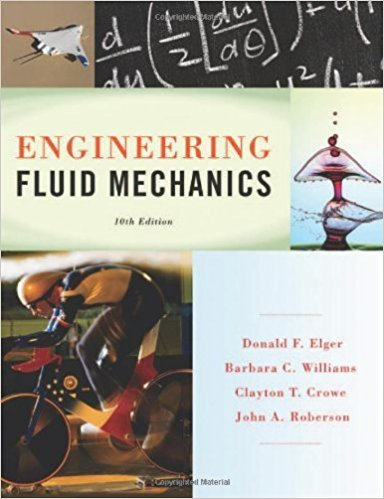> > > > Problem 16.8

# Compare and contrast the integral-form of the continuity equation (Eq. 5.28), p. 183## Problem 16.8 Chapter 16

Engineering Fluid Mechanics | 10th Edition

• 2901 Step-by-step solutions solved by professors and subject experts
• Get 24/7 help from StudySoup virtual teaching assistantsEngineering Fluid Mechanics | 10th Edition

4 5 0 414 Reviews
12
5
Problem 16.8

Compare and contrast the integral-form of the continuity equation (Eq. 5.28), p. 183, 5.3 with the PDE form of the continuity equation (Eq. 16.36) p. 6l4, 16.3. Address the following questions. a. Are the units and dimensions the same? Or different? b. How do the physics compare? What is the same? What is different? c. How do the derivations compare? What is the same? What is different? d. When would you want to apply the integral-form of the continuity equation (Chapter 5)? When would you want to apply the PDE-form of the continuity equation (Chapter 16)?

Step-by-Step Solution:
Step 1 of 3

PHYS 221 General Physics II 01/23/17 Dielectrics, Capacitors Lecture 4 Spring 2016 Electric field, electric potential Potential DiffereDevice is used for*independent of path Last Lecture electrocardiograms*independent of test charge • Electric...

Step 2 of 3

Step 3 of 3

##### ISBN: 9781118164297

Unlock Textbook Solution

Compare and contrast the integral-form of the continuity equation (Eq. 5.28), p. 183

×
Get Full Access to Engineering Fluid Mechanics - 10 Edition - Chapter 16 - Problem 16.8

Get Full Access to Engineering Fluid Mechanics - 10 Edition - Chapter 16 - Problem 16.8

I don't want to reset my password

Need help? Contact support

Need an Account? Is not associated with an account
We're here to help